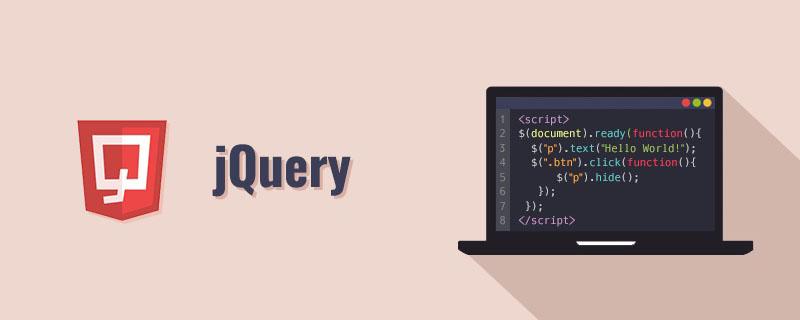\$(“#”)是指选择器选择带有id的元素，例如<input type=”text” id=”a”/> 就可以用\$(“#id”).val()来获取对应的数据值，而里面有加号的情况一般是有变量，在函数封装时常用，例如:下面的小demo

```<script src="jquery-1.8.3.js"></script>
<body>
<input type="text" value="a1" id="a1"><br/>
<input type="text" value="a2" id="a2"><br/>
<input type="text" value="a3" id="a3"><br/>
<input type="text" value="a4" id="a4"><br/>
<input type="text" value="a5" id="a5"><br/>
<input type="button" value="点击测试" οnclick="test_()">
</body>
<script>
function test_() {
var a1=\$("#a1").val();
for(var i=1;i<=5;i++) {
//这里＋只是一个连接的作用，总得来说就是jquery的选择器，没有区别的说法
var a=\$("#a"+i).val();